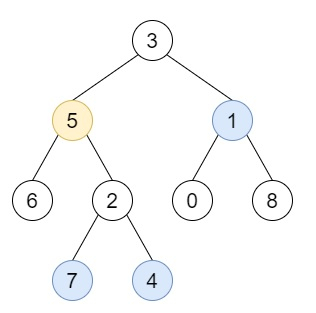# All Nodes Distance K in Binary Tree in C++

Suppose we have a binary tree, a target node, and a one value K. We have to find a list of the values of all nodes that have a distance K from the target node.

So, if the input is like root = [3,5,1,6,2,0,8,null,null,7,4], target = 5, K = 2, then the output will be [7,4,1], this is because the nodes that are a distance 2 from the target node have values 7, 4, and 1.To solve this, we will follow these steps −

• Define a function dfs(), this will take node, pa initialize it with NULL,

• if node is null, then −

• return

• parent[node] := pa

• dfs(left of node, node)

• dfs(right of node, node)

• From the main method do the following −

• Define an array ans

• dfs(root)

• Define one queue q for (node, value) pair

• insert { target, 0 } into q

• Define one set called visited

• insert target into visited

• while (not q is empty), do −

• Define one pair p := first element of q

• delete element from q

• level := second element of temp

• node = first element of temp.

• if level is same as k, then −

• insert value of node at the end of ans

• if left of node is not null and level + 1 <= k and left of node is not visited, then

• insert {left of node, level + 1 }) into q

• insert left of node into visited set

• if right of node is not null and level + 1 <= k and right of node is not visited, then

• insert {right of node, level + 1 }) into q

• insert right of node into visited set

• if parent[node] is not NULL and level + 1 <= k and parent[node] is not visited, then −

• insert { parent[node], level + 1 } into q

• insert parent[node] into visited

• return ans

## Example

Let us see the following implementation to get a better understanding −

Live Demo

#include <bits/stdc++.h>
using namespace std;
void print_vector(vector<int> v){
cout << "[";
for(int i = 0; i<v.size(); i++){
cout << v[i] << ", ";
}
cout << "]"<<endl;
}
class TreeNode{
public:
int val;
TreeNode *left, *right;
TreeNode(int data){
val = data;
left = NULL;
right = NULL;
}
};
void insert(TreeNode **root, int val){
queue<TreeNode*> q;
q.push(*root);
while(q.size()){
TreeNode *temp = q.front();
q.pop();
if(!temp->left){
if(val != NULL)
temp->left = new TreeNode(val);
else
temp->left = new TreeNode(0);
return;
}else{
q.push(temp->left);
}
if(!temp->right){
if(val != NULL)
temp->right = new TreeNode(val);
else
temp->right = new TreeNode(0);
return;
}else{
q.push(temp->right);
}
}
}
TreeNode *make_tree(vector<int> v){
TreeNode *root = new TreeNode(v);
for(int i = 1; i<v.size(); i++){
insert(&root, v[i]);
}
return root;
}
class Solution {
public:
map <TreeNode*, TreeNode*> parent;
void dfs(TreeNode* node, TreeNode* pa = NULL){
if (!node)
return;
parent[node] = pa;
dfs(node->left, node);
dfs(node->right, node);
}
vector<int> distanceK(TreeNode* root, TreeNode* target, int k) {
vector<int> ans;
parent.clear();
dfs(root);
queue<pair<TreeNode*, int> > q;
q.push({ target, 0 });
set<TreeNode*> visited;
visited.insert(target);
while (!q.empty()) {
pair<TreeNode*, int> temp = q.front();
q.pop();
int level = temp.second;
TreeNode* node = temp.first;
if (level == k) {
ans.push_back(node->val);
}
if ((node->left && node->left->val != 0) && level + 1 <= k && !visited.count(node->left)) {
q.push({ node->left, level + 1 });
visited.insert(node->left);
}
if ((node->right && node->right->val != 0) && level + 1 <= k && !visited.count(node->right)){
q.push({ node->right, level + 1 });
visited.insert(node->right);
}
if (parent[node] != NULL && level + 1 <= k && !visited.count(parent[node])) {
q.push({ parent[node], level + 1 });
visited.insert(parent[node]);
}
}
return ans;
}
};
main(){
Solution ob;
vector<int> v = {3,5,1,6,2,0,8,NULL,NULL,7,4};
TreeNode *root = make_tree(v);
TreeNode *target = root->left;
print_vector(ob.distanceK(root, target, 2));
}

## Input

{3,5,1,6,2,0,8,NULL,NULL,7,4}

## Output

[7, 4, 1, ]